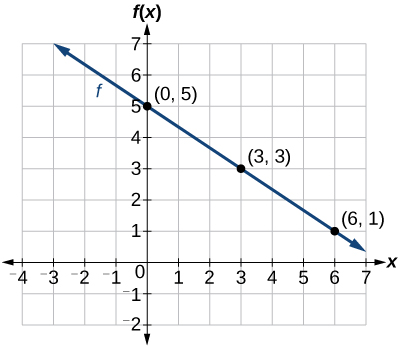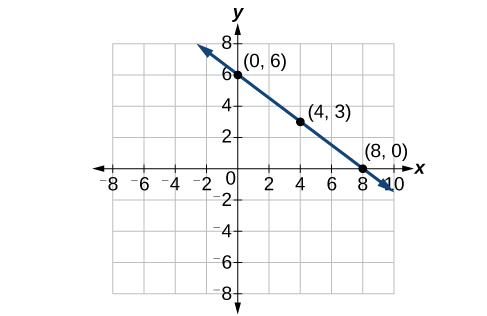# 4.1 Linear functions  (Page 7/27)

 Page 7 / 27

Is the initial value always provided in a table of values like [link] ?

No. Sometimes the initial value is provided in a table of values, but sometimes it is not. If you see an input of 0, then the initial value would be the corresponding output. If the initial value is not provided because there is no value of input on the table equal to 0, find the slope, substitute one coordinate pair and the slope into $\text{\hspace{0.17em}}f\left(x\right)=mx+b,\text{\hspace{0.17em}}$ and solve for $\text{\hspace{0.17em}}b.$

A new plant food was introduced to a young tree to test its effect on the height of the tree. [link] shows the height of the tree, in feet, $\text{\hspace{0.17em}}x\text{\hspace{0.17em}}$ months since the measurements began. Write a linear function, $\text{\hspace{0.17em}}H\left(x\right),$ where $\text{\hspace{0.17em}}x\text{\hspace{0.17em}}$ is the number of months since the start of the experiment.

 x 0 2 4 8 12 H ( x ) 12.5 13.5 14.5 16.5 18.5

$H\left(x\right)=0.5x+12.5$

## Graphing linear functions

Now that we’ve seen and interpreted graphs of linear functions, let’s take a look at how to create the graphs. There are three basic methods of graphing linear functions. The first is by plotting points and then drawing a line through the points. The second is by using the y- intercept and slope. And the third method is by using transformations of the identity function $\text{\hspace{0.17em}}f\left(x\right)=x.$

## Graphing a function by plotting points

To find points of a function, we can choose input values, evaluate the function at these input values, and calculate output values. The input values and corresponding output values form coordinate pairs. We then plot the coordinate pairs on a grid. In general, we should evaluate the function at a minimum of two inputs in order to find at least two points on the graph. For example, given the function, $\text{\hspace{0.17em}}f\left(x\right)=2x,$ we might use the input values 1 and 2. Evaluating the function for an input value of 1 yields an output value of 2, which is represented by the point $\text{\hspace{0.17em}}\left(1,2\right).\text{\hspace{0.17em}}$ Evaluating the function for an input value of 2 yields an output value of 4, which is represented by the point $\text{\hspace{0.17em}}\left(2,4\right).\text{\hspace{0.17em}}$ Choosing three points is often advisable because if all three points do not fall on the same line, we know we made an error.

Given a linear function, graph by plotting points.

1. Choose a minimum of two input values.
2. Evaluate the function at each input value.
3. Use the resulting output values to identify coordinate pairs.
4. Plot the coordinate pairs on a grid.
5. Draw a line through the points.

## Graphing by plotting points

Graph $\text{\hspace{0.17em}}f\left(x\right)=-\frac{2}{3}x+5\text{\hspace{0.17em}}$ by plotting points.

Begin by choosing input values. This function includes a fraction with a denominator of 3, so let’s choose multiples of 3 as input values. We will choose 0, 3, and 6.

Evaluate the function at each input value, and use the output value to identify coordinate pairs.

$\begin{array}{cc}\hfill x=0& \phantom{\rule{2em}{0ex}}f\left(0\right)=-\frac{2}{3}\left(0\right)+5=5⇒\left(0,5\right)\hfill \\ \hfill x=3& \phantom{\rule{2em}{0ex}}f\left(3\right)=-\frac{2}{3}\left(3\right)+5=3⇒\left(3,3\right)\hfill \\ \hfill x=6& \phantom{\rule{2em}{0ex}}f\left(6\right)=-\frac{2}{3}\left(6\right)+5=1⇒\left(6,1\right)\hfill \end{array}$

Plot the coordinate pairs and draw a line through the points. [link] represents the graph of the function $\text{\hspace{0.17em}}f\left(x\right)=-\frac{2}{3}x+5.$The graph of the linear function   f ( x ) = − 2 3 x + 5.

Graph $\text{\hspace{0.17em}}f\left(x\right)=-\frac{3}{4}x+6\text{\hspace{0.17em}}$ by plotting points.## Graphing a function using y- Intercept and slope

Another way to graph linear functions is by using specific characteristics of the function rather than plotting points. The first characteristic is its y- intercept, which is the point at which the input value is zero. To find the y- intercept, we can set $\text{\hspace{0.17em}}x=0\text{\hspace{0.17em}}$ in the equation.

root under 3-root under 2 by 5 y square
The sum of the first n terms of a certain series is 2^n-1, Show that , this series is Geometric and Find the formula of the n^th
cosA\1+sinA=secA-tanA
why two x + seven is equal to nineteen.
The numbers cannot be combined with the x
Othman
2x + 7 =19
humberto
2x +7=19. 2x=19 - 7 2x=12 x=6
Yvonne
because x is 6
SAIDI
what is the best practice that will address the issue on this topic? anyone who can help me. i'm working on my action research.
simplify each radical by removing as many factors as possible (a) √75
how is infinity bidder from undefined?
what is the value of x in 4x-2+3
give the complete question
Shanky
4x=3-2 4x=1 x=1+4 x=5 5x
Olaiya
hi can you give another equation I'd like to solve it
Daniel
what is the value of x in 4x-2+3
Olaiya
if 4x-2+3 = 0 then 4x = 2-3 4x = -1 x = -(1÷4) is the answer.
Jacob
4x-2+3 4x=-3+2 4×=-1 4×/4=-1/4
LUTHO
then x=-1/4
LUTHO
4x-2+3 4x=-3+2 4x=-1 4x÷4=-1÷4 x=-1÷4
LUTHO
A research student is working with a culture of bacteria that doubles in size every twenty minutes. The initial population count was  1350  bacteria. Rounding to five significant digits, write an exponential equation representing this situation. To the nearest whole number, what is the population size after  3  hours?
v=lbh calculate the volume if i.l=5cm, b=2cm ,h=3cm
Need help with math
Peya
can you help me on this topic of Geometry if l help you
litshani
( cosec Q _ cot Q ) whole spuare = 1_cosQ / 1+cosQ
A guy wire for a suspension bridge runs from the ground diagonally to the top of the closest pylon to make a triangle. We can use the Pythagorean Theorem to find the length of guy wire needed. The square of the distance between the wire on the ground and the pylon on the ground is 90,000 feet. The square of the height of the pylon is 160,000 feet. So, the length of the guy wire can be found by evaluating √(90000+160000). What is the length of the guy wire?
the indicated sum of a sequence is known as
how do I attempted a trig number as a starter
cos 18 ____ sin 72 evaluateByByBy Alec MoffitBy Richley CrapoBy RhodesBy OpenStaxBy OpenStaxBy OpenStaxBy Joanna SmithbackBy Sandhills MLTBy Megan EarhartBy OpenStax